Share

# Constructing a Quadrilateral - When the Lengths of Four Sides and a Diagonal Are Given

#### notes

We shall explain this construction through an example .

Example : Construct a quadrilateral PQRS where PQ = 4 cm,QR = 6 cm, RS = 5 cm, PS = 5.5 cm and PR = 7 cm.
Solution:
A rough sketch will help us in visualising the quadrilateral.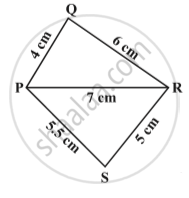Step 1 - From the rough sketch, it is easy to see that ∆PQR can be constructed using SSS construction condition. Draw ∆PQR in fig.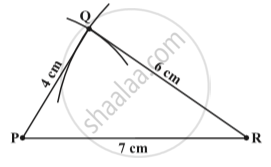Step 2 -  Now, we have to locate the fourth point S. This ‘S’ would be on the side opposite to Q with reference to PR. For that, we have two measurements.
S is 5.5 cm away from P. So, with P as centre, draw an arc of radius 5.5 cm. (The point S is somewhere on this arc)fig.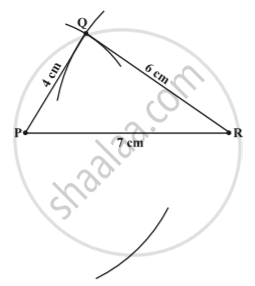Step 3:  S is 5 cm away from R. So with R as centre, draw an arc of radius 5 cm (The point S is somewhere on this arc also)in following fig.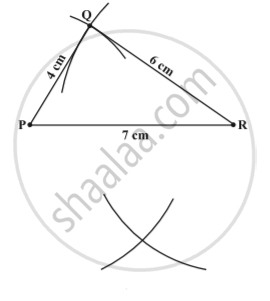Step 4 :  S should lie on both the arcs drawn. So it is the point of intersection of the two arcs. Mark S and complete PQRS. PQRS is the required quadrilateral in following fig .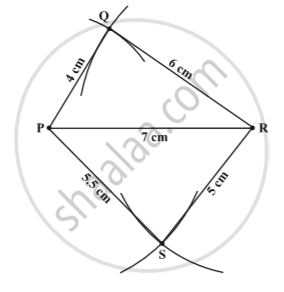### Shaalaa.com

Quadrilateral with 4 sides & 1 diagonal [00:08:40]
S
0%

S